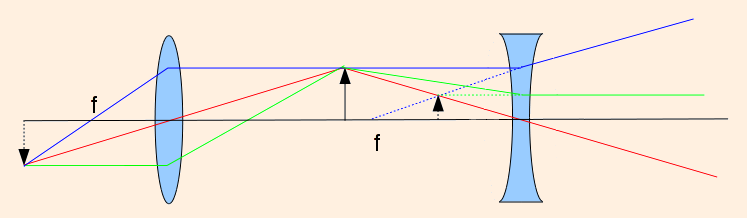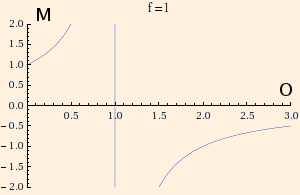### Optics

1. In optics,

• I is the image distance, O is the object distance, f is the focal length and R is the radius of curvature of a spherical lens or mirror.
• R is positive for lenses (and negative for mirrors) if the center of curvature is on the opposite side of the light from the object.
• For a flat surface, R = ∞.
• The magnification M = - I / O, which also equals - image size / object size. If M < 0, the image is inverted.

I and f are positive for lenses (and negative for mirrors) if they are on the opposite side of the lens or mirror from the light from the object:converging lens diverging lens I > 0 I < 0 Renter > 0 Renter < 0 Rexit < 0 Rexit > 0 f > 0 f < 0 M < 0 M > 0 real image virtual image

2. 1 / I + 1 / O = 1 / f.
This is called the thin lens equation.
Combining it with the definition of magnification, we find that
M = f / (f - O)This means that for negative f (diverging lenses and convex mirrors), 0 < M < 1.

It also means that for positive f (converging lenses and concave mirrors),

• if O < f, M > 1;
• as O → f -, M → ∞;
• for O > f, M < 0;
• as O → f +, M → -∞;
• when O = 2 f, M = -1; and
• as O → ∞, M → 0.

3. 1 / f = 2 / R for a mirror.
4. 1 / f = (n - 1) (1 / Renter - 1 / Rexit) for a lens in air.
This is called the lens maker's equation.
If f in measured in meters, s = 1/f is the lens strength measured in diopters.

For compound lenses, the strengths are additive.

5. For light refracting from medium 1 to medium 2 through a spherical surface of radius R,
n2 / I + n1 / O = (n2 - n1) / R.

 (prev) (top) (next)